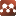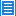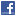## Number Theory## Sums of Powers of the Terms of Lucas Sequences with Indices in Arithmetic Progression

Authors: Kunle Adegoke

We evaluate the sums $\sum_{j=0}^k{u_{rj+s}^{2n}\,z^j}$, $\sum_{j=0}^k{u_{rj+s}^{2n-1}\,z^j}$ and $\sum_{j=0}^k{v_{rj+s}^{n}\,z^j}$, where $r$, $s$ and $k$ are any integers, $n$ is any nonnegative integer, $z$ is arbitrary and $(u_n)$ and $(v_n)$ are the Lucas sequences of the first kind and of the second kind, respectively. As natural consequences we obtain explicit forms of the generating functions for the powers of the terms of Lucas sequences with indices in arithmetic progression. This paper therefore extends the results of P.~Sta\u nic\u a who evaluated $\sum_{j=0}^k{u_{j}^{2n}\,z^j}$ and $\sum_{j=0}^k{u_{j}^{2n-1}\,z^j}$; and those of B. S. Popov who obtained generating functions for the powers of these sequences.

Comments: 5 Pages.

Download: PDF

### Submission history

[v1] 2019-04-26 01:20:05

Unique-IP document downloads: 0 times

Vixra.org is a pre-print repository rather than a journal. Articles hosted may not yet have been verified by peer-review and should be treated as preliminary. In particular, anything that appears to include financial or legal advice or proposed medical treatments should be treated with due caution. Vixra.org will not be responsible for any consequences of actions that result from any form of use of any documents on this website.

Add your own feedback and questions here:
You are equally welcome to be positive or negative about any paper but please be polite. If you are being critical you must mention at least one specific error, otherwise your comment will be deleted as unhelpful.

comments powered by Disqus